Area and Perimeter

Area and perimeter are measuring parameters related to various two-dimensional figures like triangle, rectangle, square etc.

Area

Total space enclosed by the boundary of a plane figure is called the area of that particular figure.
In another words, the area of a figure is a measure associated with the part of plane enclosed in the figure. Area is measured in square unit like, square metre, square centimetre etc.

For example:  If length (l) of a rectangle is 5 cm and breadth (b) is 4 cm, then area of rectangle is given as: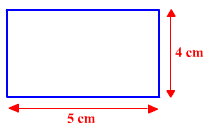Area of rectangle => l x b = 5 x 4 = 20 sq cm

Perimeter

Perimeter is the length of border around any enclosed plane. Therefore, sum of the sides of a plane figure is the perimeter of that particular figure.
Unit of perimeter is same as the unit of sides of a given figure.
Like meter, centimetre etc.

For example: If the sides of a triangle are 2cm, 8 cm and 4 cm, respectively, then perimeter of that particular triangle is given as :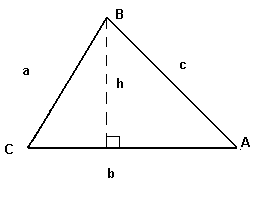Here a= 2 cm , b = 8 cm and c = 4 cm

Perimeter of that particular triangle is :
2 cm + 8 cm + 4 cm = 14 cm

Part – I: Triangles

Angles and Sides:

1. The sum of any two sides of a triangle is greater than the third side.

2. The line joining the mid-point of a side of a triangle to the opposite vertex is called the median. The median of a triangle divides it into two triangles of the same area.

3. The point of intersection of the 3 medians of a triangle is called its centroid. The centroid divides each of the medians in the ratio 2: 1.

4. The perimeter of a triangle of sides of length a, b and c is (a + b + c).

Question: In triangle PQR length of the side QR is less than twice the length of the side PQ by 2 cm. Length of the side PR exceeds the length of the side PQ by 10 cm. The perimeter is 40 cm. The length of the smallest side of the triangle PQR is:

Solution:

QR = 2PQ – 2 and PR = PQ + 10

PQ + QR + PR = 40

=>   PQ + 2PQ - 2 + PQ +10 = 40

=>   4PQ = 32

PQ = 8 cm which is the smallest side of the triangle.

Question: In an isosceles triangle ABC,A = 900, AL is drawn perpendicular to BC. FindBAL.

Solution: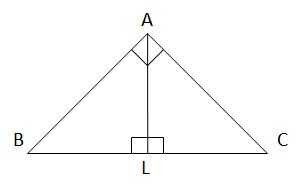A +B +C = 1800B =C = (1800 – 900) / 2 = 450BAL +BLA +B = 1800

=>BAL = 1800 – 900 – 450 = 450

Area:

1. Area of a triangle = Base x Height / 2.

2. Area of a triangle is s(s – a)(s – b)(s – c) where s = semi perimeter = (a + b + c) / 2.

3. The area of the triangle formed by joining the mid-points of the sides of a given triangle is one-fourth of the area of the given triangle.

Question: Triangle ABC is inscribed in a square of side 20 cm. Find the area of the triangle.

Solution:

From the figure, we can see that

Base = 20 cm, Height = 20 cm.

Thus, area of the triangle = 20 x 20 / 2 = 200 cm2

Question: What is the height of the triangle?

I.  The area of the triangle is 20 times its base.

II. Perimeter of the triangle = Perimeter of a square of side 10 cm.

Options:

A.   I alone sufficient while II alone not sufficient to answer

B.   II alone sufficient while I alone not sufficient to answer

C.   Either I or II alone sufficient to answer

D.   Both I and II are not sufficient to answer

E.    Both I and II are necessary to answer

Solution:

I.  1/2 x B x H = 20 x B     or, H = 40

I alone gives the answer. II. Gives, perimeter of the triangle = 40 cm but this does not give the height of the triangle.

Types and Properties:

1.    Equilateral Triangle: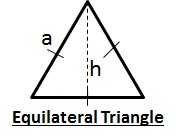a)    The angles of the triangle are equal to 600 each.

b)    All the sides of the triangle are equal.

c)    Altitude of an equilateral triangle = 3a / 2

d)    Area of an equilateral triangle = 3a2/4

2.    Isosceles Triangle: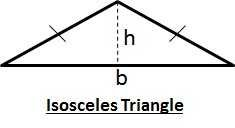a)    The two base angles of the triangle are equal.

b)    Two sides of the triangle are equal.

3.    Scalene Triangle: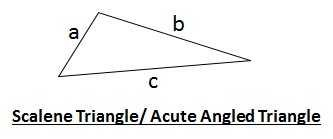a)    No two angles have the same value.

b)    No two sides have the same length.

4.    Acute-angled Triangle:

a)     All angles of the triangle are lesser than 900.

5.    Right-angled Triangle: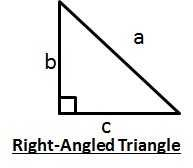a)    One angle of the triangle is 900 while the other 2 are lesser than 900 each.

b)    a2 = b2 + c2

Types:

1. TrapeziumA quadrilateral having exactly one pair of parallel sides is called a trapezium.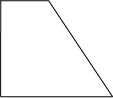2. Isosceles Trapezium: A trapezium whose non-parallel sides are equal in length is called an isosceles trapezium.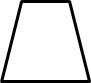3. Parallelogram: A quadrilateral is said to be a parallelogram if both pairs of its opposite sides are parallel.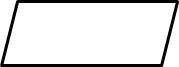4. Rhombus: A parallelogram having all sides equal is called a rhombus.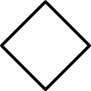5. Rectangle: A quadrilateral in which each angle is a right angle is called a rectangle.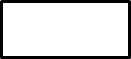6. Square: A square is a quadrilateral in which all sides are equal and each angle measures 900.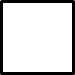Angles and Sides:

1. The diagonals of a parallelogram bisect each other.

2. Perimeter of a rectangle = 2(Length + Breadth)

3. Perimeter of a square = 4 x length of a side

4. Each diagonal of a parallelogram divides it into triangles of the same area.

5. The diagonals of a rectangle are equal and bisect each other.

6. The diagonals of a square are equal and bisect each other at right angles.

7. The diagonal of a square of side a = a2.

8. The diagonals of a rhombus are unequal and bisect each other at right angles.

9. The sum of angles of a quadrilateral is 360 °

Question: What is the smaller angle of a parallelogram?

I.  Ratio of the angles of a triangle is 3:4:5 and the larger angle of the parallelogram is 340 greater than the largest angle of the triangle.

II. Larger angle of the parallelogram is 38° more than its smaller angle.

A.   I alone sufficient while II alone not sufficient to answer

B.   II alone sufficient while I alone not sufficient to answer

C.   Either I or II alone sufficient to answer

D.   Both I and II are not sufficient to answer

E.    Both I and II are necessary to answer

Solution:

I. The sum of the angles of a triangle is 1800. Thus, the angles can be determined and hence, larger angle of parallelogram. Larger angle + Smaller angle = 1800. So I alone is sufficient.

II. Sum and difference of angles is known. Both angles can be calculated. II alone is sufficient.

Thus, the correct answer is C.

Area:

1.    Area of a rectangle = (Length x Breadth).

2.    Area of a square = (side)2 = ½ x (diagonal)2.

3.    Area of parallelogram = (Base x Height).

4.    Area of a rhombus = ½ x (Product of diagonals).

5.    Area of a trapezium = ½ x (sum of parallel sides) x distance between them.

Question: An error of 2% in excess is made while measuring the side of a square. Calculate the percentage of error in the calculated area of the square.

Solution:

Let the actual length of side be x. Then measured length = 1.02x.

Actual area = x2. Measured area = (1.02x)2.

Error in area calculation = (1.022 – 12)x2 / x2 = (1.02 + 1)(1.02 – 1) = 2.02 x 0.02 = 0.0404

Percentage error = 0.0404 x 100% = 4.04%

Question: A rectangular park 60 m long and 40 m wide has two concrete crossroads running in the middle of the park and rest of the park has been used as a lawn. If the area of the lawn is 2109 sq. m, then what is the width of the road?

Solution: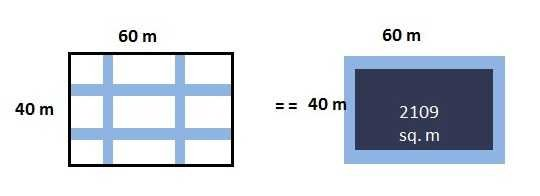Let the width of the road be x meters.

Then, (60 – 2x)(40 – 2x) = 2109

=>    2400 – 200x + 4x2 = 2109

=>    4x2 – 200x + 291 = 0

=>    x = (200 ± 188) / 8 = 1.5 or 48.5

But width of road cannot be greater than breadth of the park. Thus, width of road = 1.5m.

Part – III: Circles – Angles, Radii and Area

1.    Area of a circle =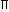R2, where R is the radius

2.    Circumference of a circle = 2R

3.    Length of an arc = 2R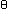/ 360, whereis the central angle.

4.    Area of a sector = ½ x (arc length x R) =R2/ 360

5.    Area of semi-circle =R2 / 2

Question: A lawn is in the shape as shown. Find the area of the lawn.

Solution: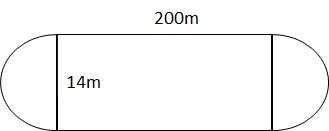The lawn is in the form of a rectangle with two semicircles on opposite ends of the width.

Area of the rectangle = 200m x 14m = 2800 sq. m

Radius of the semicircles = 14/2 m = 7 m

Area of the two semicircles = 2 x (R2 / 2) = 22 / 7 x 7 x 7 = 154 sq. m.

Total Area of the lawn = 2954 sq. m.

Question: ABCD is a square with one vertex at the center of the circle and two vertices on the circle. What is the length of the diagonal of the square if the area of the circle is 100 square cm?

Solution: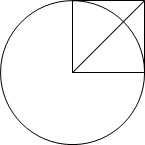Area of circle =R2 = 100 sq. cm

R2 = 100/Or, R = 10/cm

From the figure, side of square, a = Radius of circle, R = 10/cm

Diagonal of the square = a2 = 10(2/) cm